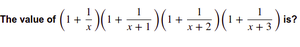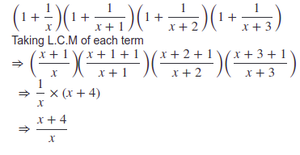# Algebra

• Last Updated : 13 Oct, 2021

12
 Question 1

Given two numbers x and y such that

1. x3 + y3 = 9

2. x + y = 3

Find the value of x4+y4

 A 2/3 B 7 C 13 D 17
Algebra
Discuss it

Question 1 Explanation:
x3+y3 = (x + y) × (x2 − xy + y2)

Putting given values of x3+y3 and (x + y)
9 = 3 × ((x+y)2 − 3xy)
= 3 × (9 − 3xy)
= 27 − 9xy

9xy = 18
xy = 2

x4 + y4 = (x2 + y2)2 - 2x2y2
= (x2 + y2)2 - 2*4
[Putting value of xy]
= ((x + y)2 - 2xy)2 - 2*4
[Putting values of (x+y) and xy]
= (9 - 4)2 - 2*4
= 17
 Question 2

The value of x+1/2x is given as 2, then find the value of 8x3+1/x3

 A 40/3 B 20/7 C 28 D 40
Algebra
Discuss it

Question 2 Explanation:

x3 + 1/8x3 + 3.x.1/2x(x + 1/2x) = (x+1/2x)3

x3 + 1/8x3 + 3 = 23

x3 + 1/8x3 = 5

8x3 + 1/x3 = 5.8 = 40

 Question 3

If x takes only real numbers. For what value of x, the value of the expression: 4−6x−x2 will be maximum?

 A -4 B -3 C 0 D 3
Algebra
Discuss it

Question 3 Explanation:

Differentiate and equate to 0 6+2x =0 x=−3

 Question 4

For what value of x, the following equation: 5√x +12√x =13√x will be true?

 A -2 B 0 C 3 D None of the above
Algebra
Discuss it

Question 4 Explanation:

say x=1
17!=13

for, x=2 and 3 also not possible
x=4
52+122=132
169=169

 Question 5

If value of x = (√2 + 1 )-1⁄5 then the find the value of (x5 - 1x5)

 A 0 B -2 C 2/3 D 4
Algebra
Discuss it

 Question 6

If the value of b2 + 1b2 = 1, then find the value of b3 + 1b3

 A -1/12 B -2 C -4 D None of the above
Algebra
Discuss it

Question 6 Explanation:
E = (b + 1⁄b)(b2 - 1 + 1⁄b2)
 Question 7A 1 + 1/(x+4) B x+4 C 1/x D x+4/ x
Algebra
Discuss it

Question 7 Explanation:Question 8
If ab+bc+ac = 0, then the value ofA 6 B 2 C 3 D 0
Algebra
Discuss it

Question 8 Explanation:
Put -ac = ab + bc ; -ab = ac+ bc and -bc =ab + ac
(ab + bc + ca)/[(a+b+c)(abc)]
as, ab+bc+ca=0
= 0
 Question 9
Following instructions are to be used throughout the quiz:

Each of the questions given below consists of a statement and/or a question and two statements numbered I and II given below it. You have to decide whether the data provided in the statement(s) is/are sufficient to answer the given question.
(a) if the data in Statement I alone is sufficient to answer the question, while the data in Statement II alone is not sufficient to answer the question.
(b) if the data in Statement II alone is sufficient to answer the question, while the data in Statement I alone is not sufficient to answer the question.
(c) if the data in each Statement I and Statement II alone is sufficient to answer the question.
(d) if the data even in both Statements I and II together are not sufficient to answer the question.
(e) if the data in both Statements I and II together are necessary to answer the question.

If x,y are integers, then (x2 + y2)1/2 is an integer?
I) x2 + y2 is an integer
II) x2 - 3y2 = 0
 A A B B C C D D E E
Data Sufficiency    Algebra
Discuss it

Question 9 Explanation:
Statement 1: x2 + y2 is an integer Since x and y are integer x2+y2 can be any real number which may not be a perfect square so statement 1 alone cant prove whether (x2+y2)(½) is an integer or not. Statement 2: x2- 3y2 = 0 =>x2+y2-4y2=0 =>x2+y2=4y2 => (x2+y2)(½)= 2y Which is an integer because y is an integer.
 Question 10
4/15 of 5/7 of a number is greater than 4/9 of 2/5 of the same number by 8. What is half of that number?
 A 630 B 63 C 315 D 105
Algebra
Discuss it

Question 10 Explanation:
Let the number be x. Then, 4/15 of 5x/7 = 4/9 of 2x/5 + 8 4/15 * 5x/7 = 4/9 * 2x/5 + 8 4x/21 = 8x/45 + 8 x/21 = 2x/45 + 2 x/21 = (2x + 90)/45 45x = 21(2x + 90) 45x = 42x + 90*21 3x = 90*21 x = 30*21 = 630. Half of the number will be x/2 x/2 = 630/2 = 315.
There are 19 questions to complete.
12
My Personal Notes arrow_drop_up
Related Articles